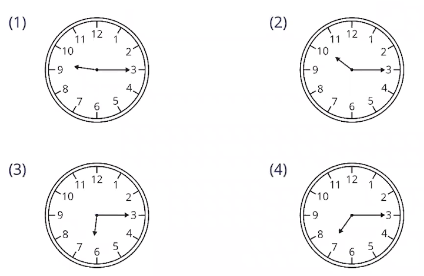Study P4 Mathematics Maths - Time - Geniebook# Time

In this article, we will be learning about the conversion of time and the 24-hour clock. The lesson objectives are:

1. Conversion of time
2. 24-h clock

## Topic Recap:

### Conversion of Time

We can express time in hours and minutes to minutes.

Examples:

1. $$1 \text{ h } = 60 \text{ min}$$
2. \begin{align}\\ 2 \text{ h } &= 2 \times 60 \text{ min}\\ &= 120 \text{ min} \end{align}
1. \begin{align}\\ 3 \text{ h } 20 \text{ min } &= 180 \text{ min }+ 20 \text{ min }\\ &= 200 \text{ min } \end{align}

We can also express time in minutes to hours and minutes.

Examples:

1. $$180 \text{ min } = 3 \text{ h } 0 \text{ min }$$
2. \begin{align}\\ 400 \text{ min } &= 360 \text{ min }+ 40 \text{ min }\\ &= 6 \text{ h } 40 \text{ min } \end{align}

## 1. Conversion Of Time

$$1 \text{ minute } = 60 \text{ seconds}$$

To express time from minutes to seconds, we multiply the number of minutes by 60.

To express time from seconds to minutes, we divide the number of seconds by 60.

$$1 \text{ hour} = 60 \text{ minutes}$$

To express from hours to minutes, we multiply the number of hours by 60.

To express from minutes to hours, we divide the number of minutes by 60.

Question 1:

Express the following time in seconds.

1. 2 min = __________ s
2. 2 h = __________ s
3. $$\frac {1}{4}$$ h = __________ s

Solution:

1. \begin{align}\\ 2 \text{ min } &= 2 \times 60\\ &= 120 \text{ s} \end{align}

120 s

1. \begin{align}\\[2ex] 2 \text{ h } &= 2 \times 60\\ &= 120 \times 60\\ &= 7200 \text{ s} \end{align}

7200 s

1.  $$\frac {1}{4} \text{ h}$$

1 h = 60 min

$$\frac {1}{4}$$h   = 60 min ÷ 4

= 15 min

15 min = 15 60 s

15    = 900 s

900 s

Question 2:

Express the following time in seconds.

5 min = __________ s

Solution:

5 min = 5 × 60 s

= 300 s

300 s

Question 3:

Express the following time in seconds.

45 min = __________ s

Solution:

45 min = 45 × 60 s

= 45 × 6 × 10

= 2700 s

2700 s

Question 4:

Express the following time in seconds.

$$1\frac {1}{2}$$ h = __________ s

Solution:

1 h = 3600 s

$$\frac {1}{2}$$= 30 min

= 30 × 60

= 1800 s

$$1\frac {1}{2}$$= 3600 s + 1800 s

= 5400 s

5400 s

Question 5:

Express the following time in minutes or hours and minutes.

1. 180 s = __________ min
2. 3000 s = __________ min
3. 4500 s = __________ h __________ min

Solution:

1. 180 s = 180 ÷ 60

= 3 min

3 min

1. 3000 s = 3000 ÷ 60

= 50 min

50 min

1. 4500 s = 4500 ÷ 60

= 75 min

= 1 h 15 min

OR

1 h = 3600s

4500 s - 3600 s = 900 s

= 900 ÷ 60

= 15 min

Hence, 4500 s = 1 h 15 min

1 h 15 min

Question 6:

Express the following time in minutes or hours and minutes.

120 s = __________ min

Solution:

120 s

= 120 ÷ 60

= 2 min

2 min

Question 7:

Express the following time in minutes or hours and minutes.

300 s = ___________ min

Solution:

300 s

= 300 ÷ 60

= 5 min

5 min

2) 24-h clock

In the 24-hour clock, there is no need to add suffixes like am or pm. It is written in 4 digits, where the first two digits represent the hour and the last two digits represent the minutes.

Time from midnight to noon:

12-h clock = 12:00 am to 11:59 am

24-h clock = 00 00 to 11 59

Time from noon to midnight:

12-h clock = 12:00 pm to 11:59 pm

24-h clock = 12 00 to 23 59

Example:

 12-h clock 24-h clock 5:30 am 05:30 5:30 pm 17:30 10:30 am 10:30 10:30 pm 22:30 12:00 pm 12:00 12:00 am 00:00

Question 1:

Express the following time in 24-hour clock.

1. 8:50 am = __________
2. 12:20 am = __________
3. 9:10 pm = __________

Solution:

a) 8:50 am = 08 50

08 50

b) 12:20 am = 00 20

00 20

c) 9:10 pm = 21 10

21 10

Question 2:

Express the following time in 24-hour clock.

2:35 am = __________

Solution:

2:35 am = 02 35

Question 3:

Express the following time in 24-hour clock.

11:15 pm = __________

Solution:

11:15 pm = 23 15

23 15

Question 4:

Which of the following clocks shows the time 19:15?Solution:

19 15 is 7:15 pm in the evening.

(4)

Question 5:

Express the following time in 12-hour clock.

1. 03 30 = __________
2. 12 50 = __________
3. 17 45 = __________

Solution:

1.  03 30 = 3:30 am

1. 12 50 = 12:50 pm

1. 17 45 = 5:45 pm

Question 6:

Express the following time in 12-hour clock.

00 10 = __________

Solution:

00 10 = 12:10 am

12:10 am

Question 7:

Express the following time in 12-hour clock.

20 15 = __________

Solution:

20 15 = 8:15 pm

8:15 pm

Continue Learning
Multiplication Whole Numbers
Multiplication And Division Decimals
Model Drawing Strategy Division
Fractions Factors And Multiples
Area And Perimeter 1 Line Graphs
TimePrimaryPrimary 1Primary 2Primary 3Primary 4EnglishMaths
Multiplication
Whole Numbers
Line Graphs
Time
Multiplication And Division
Decimals
Model Drawing Strategy
Division
Fractions
Factors And Multiples
Area And Perimeter 1SciencePrimary 5Primary 6SecondarySecondary 1Secondary 2Secondary 3Secondary 4